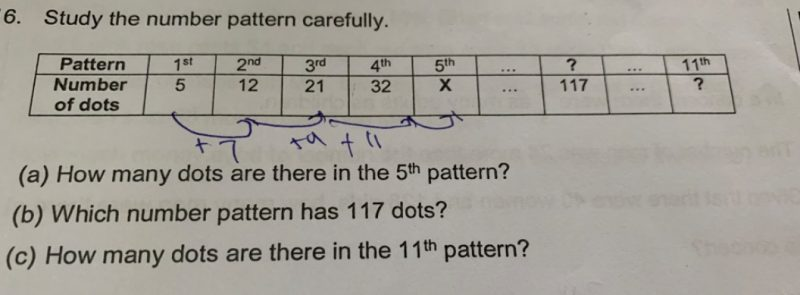# Question1 Answer

# Answer

(a)
5 + 7 + 9 + 11 + 13 = 45

(b)
Pattern —
5th : 45
6th : 60
7th : 77
8th : 96
9th : 117

(c)
10th : 140
11th : 165

Alternatively (more suitable for secondary school level),
(a)
Using an^2 + bn,
a + b = 5
4a + 2b = 12
2a + b = 6
a = 1, b = 4
5^2 + 4 x 5 = 45

(b)
n^2 + 4n – 117 = 0
(n – 9)(n + 13 ) = 0
n = 9

(c)
11^2 + 11 x 4 = 165

Ans : (a) 45 dots; (b) 9th pattern; (c) 165 dots.

0 Replies 1 Like ✔Accepted Answer